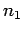Next: Statistical mechanics Up: Probability theory Previous: The Gaussian distribution

## The central limit theorem

Now, you may be thinking that we got a little carried away in our discussion of the Gaussian distribution function. After all, this distribution only seems to be relevant to two-state systems. In fact, as we shall see, the Gaussian distribution is of crucial importance to statistical physics because, under certain circumstances, it applies to all systems.

Let us briefly review how we obtained the Gaussian distribution function in the first place. We started from a very simple system with only two possible outcomes. Of course, the probability distribution function (for) for this system did not look anything like a Gaussian. However, when we combined very many of these simple systems together, to produce a complicated system with a great number of possible outcomes, we found that the resultant probability distribution function (for) reduced to a Gaussian in the limit as the number of simple systems tended to infinity. We started from a two outcome system because it was easy to calculate the final probability distribution function when a finite number of such systems were combined together. Clearly, if we had started from a more complicated system then this calculation would have been far more difficult.

Let me now tell you something which is quite astonishing! Suppose that we start from any system, with any distribution function (for some measurable quantity). If we combine a sufficiently large number of such systems together, the resultant distribution function (for) is always Gaussian. This proposition is known as the central limit theorem. As far as Physics is concerned, it is one of the most important theorems in the whole of mathematics.

Unfortunately, the central limit theorem is notoriously difficult to prove. A somewhat restricted proof is presented in Sections 1.10 and 1.11 of Reif.

The central limit theorem guarantees that the probability distribution of any measurable quantity is Gaussian, provided that a sufficiently large number of statistically independent observations are made. We can, therefore, confidently predict that Gaussian distributions are going to crop up all over the place in statistical thermodynamics.Next: Statistical mechanics Up: Probability theory Previous: The Gaussian distribution
Richard Fitzpatrick 2006-02-02